Disclaimer: This is an example of a student written essay.
Click here for sample essays written by our professional writers.

Any scientific information contained within this essay should not be treated as fact, this content is to be used for educational purposes only and may contain factual inaccuracies or be out of date.

# A Study of the Detection of Exoplanets for the K2 Mission

 ✅ Paper Type: Free Essay ✅ Subject: Sciences ✅ Wordcount: 10921 words ✅ Published: 18th May 2020

A study of the detection of exoplanets for the k2 mission

Abstract

The Kepler space telescope was initially launched in July of 2009, with the purpose of discovering and confirming exoplanet candidates. To date 4031 have been discovered, 385 of which have been confirmed by the k2 mission which was initiated in 2014 (NASA exoplanet archives). The k2 mission is the second mission by the Kepler space telescope with the express purpose of observing fields pointed away from the Sun, along the ecliptic plane. Research aims for the internship are as follows; learn how data is processed in order to locate exoplanets. Create a program to accurately detect and model exoplanet transits, using existing modules and libraries, as well as open source code, which will be improved upon. Finally, to confirm as many discovered exoplanets as possible and attempt to locate a new undiscovered planet.

1. Introduction

## Transit photometry is an exoplanet detection method, where the flux of a star is recorded over a period of time. If a planet or planets are present, characteristic dips can be observed in the light curve at a set period known as the orbital period, from these light curves we can determine a number of parameters about the planet. The k2 data was obtained through the Mikulski Archive for Space Telescopes, which you can access through this link (MAST). These fits files contain a primary header data unit (hdu) with metadata stored in the header, which stores arrays of data in a binary fits table, which include two types of fluxes (SAP, PDCSAP). The second extension hdu consists of an image that contains the collected pixels for the target.

The report is divided into sections as follows, section 2 describes the prior processing that the light curves have undergone as described in the data release notes, that can be found in the MAST archives. Section 3 describes the steps that were taken to process the light curves, section 4 focuses on investigating possible algorithms that can be used to detect transits. Section 5 of this report describes how a unique solution can be found for the planet parameters, which are used to model the light curves in section 6. Section 7 of this report uses, Bayesian statistical inference methods in order to model probabilities for the planet’s parameters. Finally, sections 8 contains results, section 9 contains the conclusions draw from the research and section 10 contains future research plans, references are given at the very end of this report.

2. Prior Processing

All information concerning prior processing was obtained from the data release notes from this source (Andrew Vanderburg). Briefly described below are the steps taken by the k2 data processing team to process the raw light curves.

2.1 Aperture Photometry

Simple aperture photometry was carried out as follows; several different apertures of various sizes were defined; this was accomplished by using two different methods. Firstly, a circle of pixels around the star was extracted and secondly, a circle modelled by the pixel’s response function is extracted. The optimal radius was determined through trial and error to maximize photometric precision.  After which the median flux was calculated for each image, the pipeline performed background subtraction, the fluxes were summed, and the centroid position of each target was measured. Following Vanderburg & Johnson (2014), the centroid position was measured in two ways; calculating the “centre of flux,” and fitting a Gaussian to the core of the PSF. The photometry method used in the MAST data is nearly identical to the method used by Vanderburg & Johnson (2016) and Vanderburg & Johnson (2014) and remain constant throughout the k2 data processing. Please note that small changes were made to the pipeline used for the different campaigns. Please refer to the data release notes linked above for further details on data processing for specific campaigns.

2.2 Self Flat Fielding (SFF)

Kepler’s primary objective of locating transiting planets, was greatly compromised when one of the wheels responsible for the pointing mechanism malfunctioned, this left the craft with a multitude of problems, which greatly decreased the photometric precision. Thrusters were regularly fired in order to stabilise the craft which was drifting due to solar radiation pressure. Due to this corrective measure a new systematic error was introduced, if several basic assumptions are made about this motion, the systematic error can be corrected for using a method called self-flat fielding (SFF), which is outlined below.

Get Help With Your Essay

If you need assistance with writing your essay, our professional essay writing service is here to help!

Firstly, Kepler rolls in an arc, this is used to calculate an arclength for the drift. Secondly the flux can be shown to depend on image centroids, which are calculated for a relative stable star and then subtracted from the raw data. Note that unlike Vanderburg & Johnson (2014) who used each individual star’s image centroids to calculate arclength, the MAST data uses the image centroids for one particular well-behaved bright star for each campaign. The campaign was also broken into shorter divisions, as the motion of the craft is not confined to one dimension. After measuring the correlation, a linear function was fitted to the data, and then divided from the raw light curve, yielding higher quality photometry.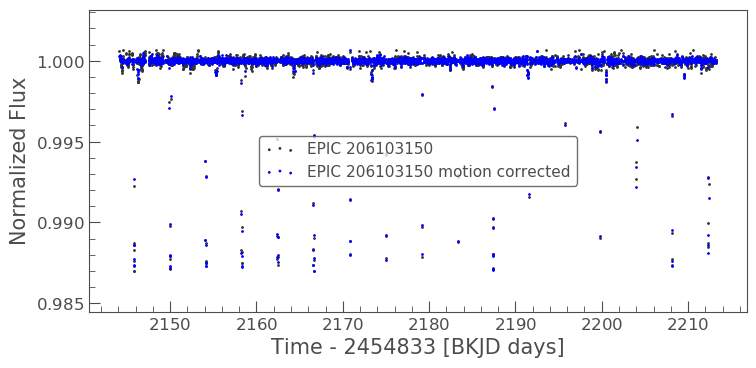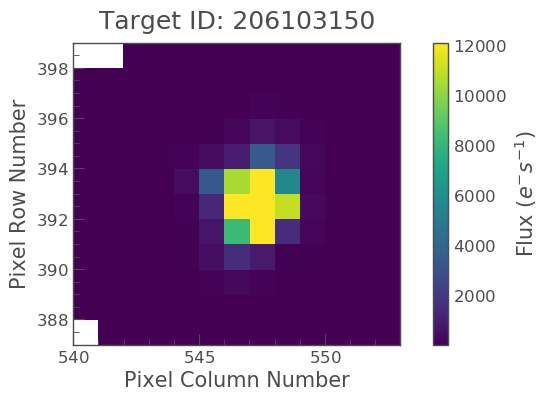Fig 1.-Image on the left:  Motion corrected curve after (SFF) for (EPIC 206103150) Image on the right: target pixel file for (EPIC 206103150)

3. Data Processing

Before commencing data processing the data release notes where obtained from the MAST archives which contained information on the campaigns and how the data was processed. The first target was selected from campaign 3, as this campaign suffers from significantly less drift, when the craft changes direction, and therefore has greater photometric precision. A search was conducted for single planet systems in NASA’s archives, only planets with masses roughly the same size or greater than Jupiter were considered, as the larger the planet the bigger the dip in light that are observed. The raw data and corrected light curves for several different systems were viewed and eventually EPIC (206103150) was selected, due to the very clear transits, and low noise/ interference. Some unusual spikes and dips in flux can be observed, which are primarily due to cosmic rays and asteroids. Initially the code was only tested on a few carefully chosen targets. The data was accessed through MAST archives, these files contain two types of fluxes, SAP flux and PDCSAP flux, the first is a simple aperture photometry flux, which is the flux after summing the calibrated pixels. The PDCSAP flux stands for pre-search data conditioned simple aperture photometry, which is the SAP flux corrected for the instrumental errors. Therefore, PDCSAP was used for all data processing, PDCSAP flux is hereafter referred to as flux. Several modules and libraries were also used from a number of different sources during the course of my internship. All sources have been cited at the end of this paper and relevant modules and libraries are included when necessary.

3.1 Removal of flagged data

The raw light curves contain several poor-quality data points due to numerous different errors including but not limited to cosmic rays, pointing adjustments, and detector glitches. which are given in the table below which can be found in Kepler’s manual archives. This flagging system was used to identify these data points, which were then removed from the light curves. Typically, between 2% and 4% of datapoints are excluded at this stage.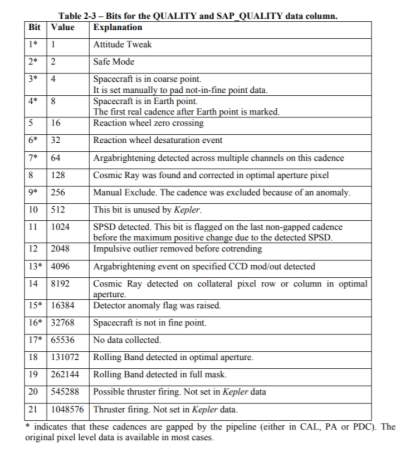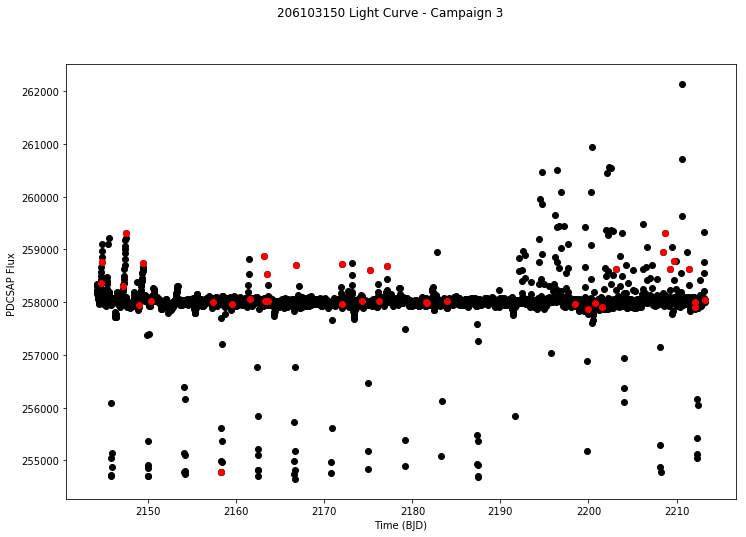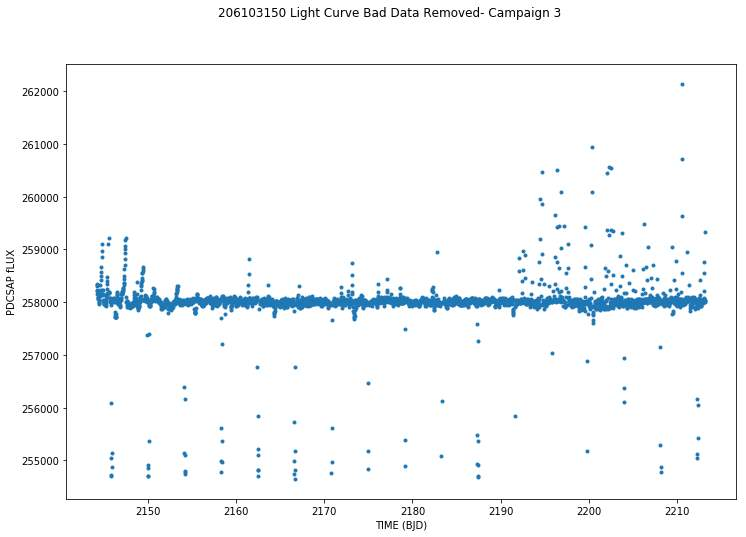Fig 2.-Top: Table with flag descriptions. Middle: Light curve with flagged data points in red. Bottom: Light curve with flagged data points removed.

3.2 Sigma clipping

In order to remove outliers, a sigma clip was used, which can be located in the open source library Lightkurve. The sigma clip works by removing any data points that lie outside set sigma thresholds. This method is very effective if the noise can be modelled as gaussian. This outlier exclusion method reduces the influence of short–timescale astrophysical noise on the correction. The number of standard deviations that should be applied, relies on the size of the data, this threshold was determined through trial and error. For upwards outliers approximately 4σ upwards outliers were removed, which are primarily caused by cosmic ray hits and asteroids. In addition to these upwards outliers, there are sparse downward outliers in the light curves. To limit the effect of these while being careful to avoid discarding exoplanet transits, a limited downward-outlier exclusion was performed. This was done by removing the two largest single-point downward excursions from each light curve. Transit events typically have durations longer than one long cadence datapoint, unless the planet’s orbital period is very short, in which case there would be many more than two transits in the 35–80 days of K2 observations (Andrew Vanderburg 2014).

3.3 Savitzky-Golay Filter

A Savitzky-Golay filter was used to remove long term trends from the data, long term trends can distort the relationship between time and flux. The module flatten() from the Lightkurve library was used, which provides a suitable filter. The Savitzky-Golay filter works by modelling the trend with a polynomial using a least-squares method. This process is known as convolution, and once the trend has been modelled, it can be removed from the data. This method is highly effective at smoothing time series like my light curve, but unfortunately there is a trade off as this method tends to over smooth sharp dips such as transits.

3.4 Remove infinite values

Cadences where the flux was NaN where then removed, NaN values would prevent further processing, as arrays with NaN values can’t be processed.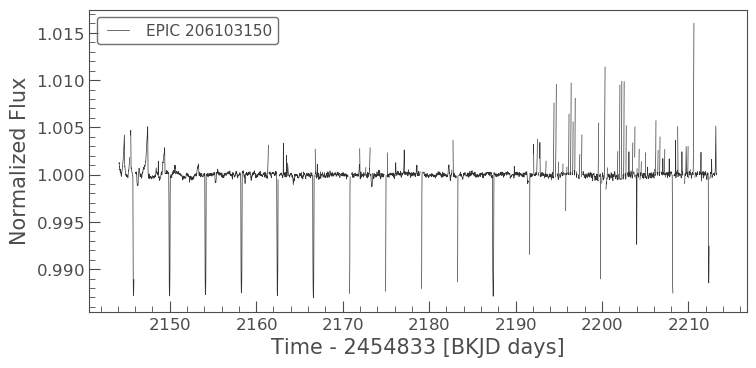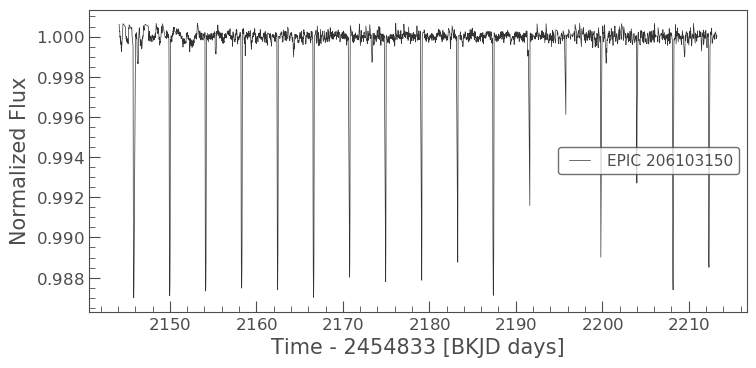Fig 3.- Top: Light curve (EPIC 206103150) before processing. Bottom: Light curve (EPIC 206103150) after processing.

4. Detect transit

4.1 Lomb-Scarple Algorithm

In order to optimise transit detection, two different algorithms were investigated; Lomb-Scarple LS & Box Least Square BLS, both of which are commonly use in the modelling of time series. The main different between the two algorithms are the type of signal they use for modelling, the LS algorithm uses a sine wave and the BLS algorithm uses a square wave, both conduct their search on a frequency grid. The LS periodogram algorithm works by transforming the data from the time domain to the frequency domain using sinusoidal functions. The LS algorithm worked as follow, plot the periodogram (Amplitude, Frequency) to check that there was a large frequency peak which could indicate that there is a large oscillation around a certain period. Then plot the periodogram in period space, which can be used to determine the period at the max power, where the max power is the period with the highest peak, which indicates the most likely true period. The light curve was then folded using a library fold from (pyke), to produce a phase folded light curve, the fit can then be investigated. When superimposing all the transits, all the data should lie on top of one another as seen in the light curves below.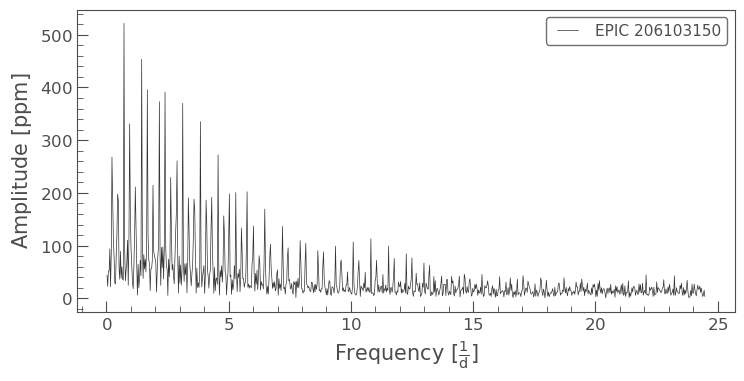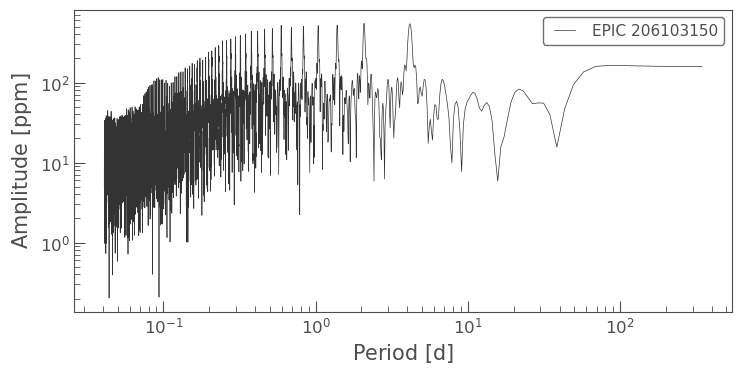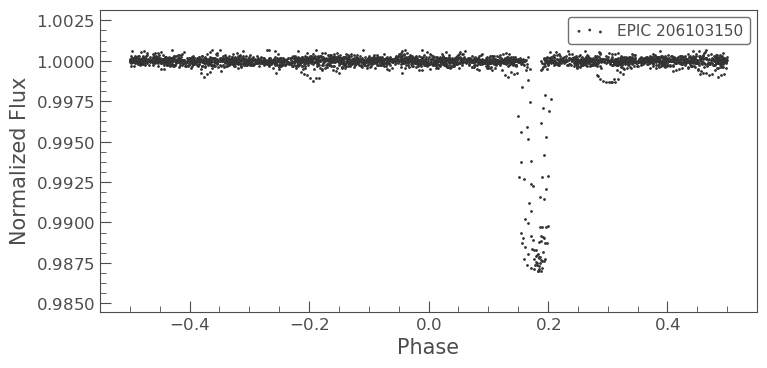Fig 4.- Top: Periodogram (Amplitude vs Frequency (EPIC 206103150)). Middle: Periodogram (Amplitude vs Period (EPIC 206103150)). Bottom: Phase folded light curve (EPIC 206103150)).

4.2 Box Least Square Algorithm

Box Least Square works by modelling the periodic transits as a square wave with four parameters, period, duration, depth and a reference point, as seen in the diagram below. A periodic box-shaped function alternate between a low and a high state, with a fixed period and phase of each periodic cycle. The Fourier transform of a light curve can be defined as a set of complex numbers one real and one imaginary for each frequency. If the total duration is T and the time resolution is t, the Fourier transform will have a minimum at a frequency of 1/T and a maximum at 1/2t, with frequency steps of 1/T. The true period of the time series can be found by plotting a power density spectrum, which is defined as the Fourier Transform of the autocorrelation function of a signal at each frequency; both the period and duration were defined as an array, then the limits of the periods to be tested, and the bin factor were set. Both were determined using trial and error. The code then runs through all the possible periods in the array. Because the light curve is not a perfect sine/cosine wave we expect to see peaks at both the true period and the harmonics, this is observed in the power density spectrum below.

After determining the true period, the transit as fitted using the box least square method as follows; the BLS function will be fitted to the data by iterating upon the epoch of mid-transit in the model and adjusting the width of the modelled transit. The width is adjusted systematically in step sizes equalling the cadence of the input data.  Before the BLS transit model is fitted to the data, the data is folded upon the orbital period and then phase binned by calculating the mean flux level within each bin interval. The data is then binned as there was a lot of data points as seen in the LS phase folded light curves, binning averages multiple data points together so that our graph has a reasonable number of points, this was accomplished by using the bin() function for the Lightkurve library. After a possible period was calculated, a graph was checked so that harmonics could be checked for. The best fit period can be seen on the period vs power graph, where the period with the max power is the true period, note the harmonics to the left and right in the graph.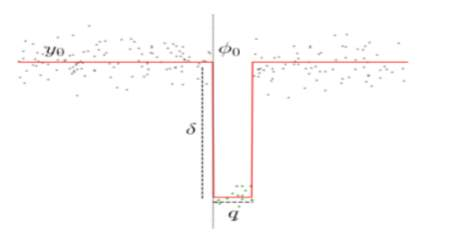Fig 5.- (Parameter used for (BLS)): The transit duration as a fraction of the period ttrans/Pttrans/P = q. The phase offset of the transit (from 0) = phi0. The difference between the out-of-transit brightness and the brightness during transit = delta. The out-of-transit brightness = y0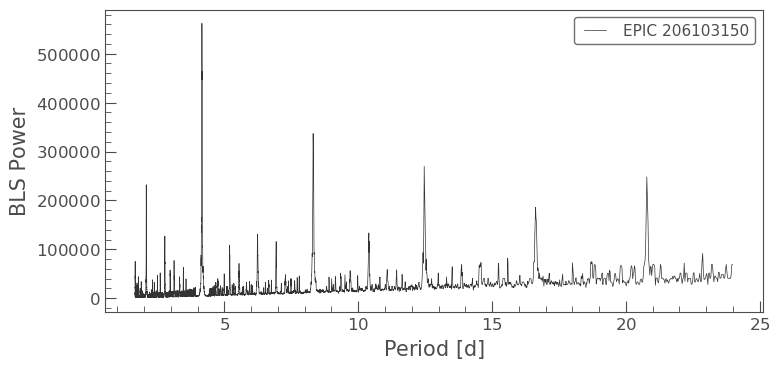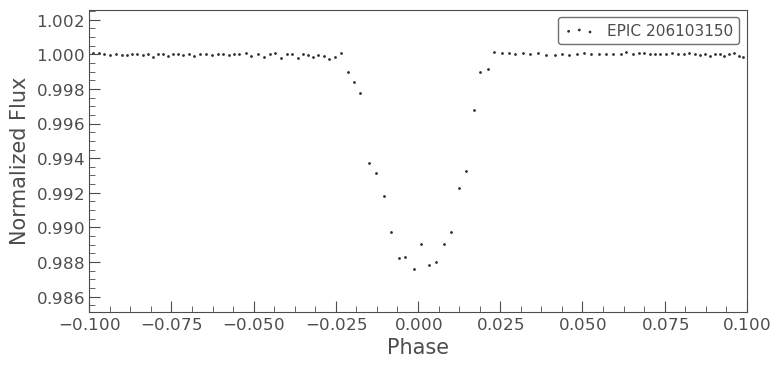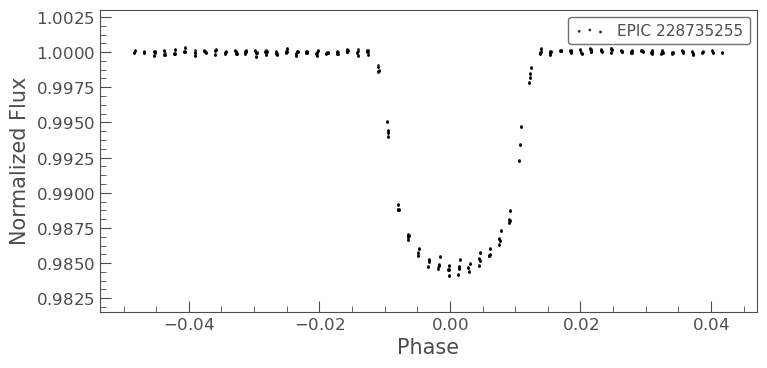Fig 6.- Top: power density spectrum (EPIC 206103150). Middle: Phase folded binned light curve (206103150). Bottom: Phase folded light curve (228735255).

4.3 Lomb-Scarple LS in comparison to Box least square BLS

There are several key differences in the performance of both algorithms, the box least square method more closely models the transits as it uses a box shaped wave instead of a sinusoidal wave. The LS unlike BLS assumes that there is only one signal causing the variation in flux, this is not always true, as there could be multiple planets in a system. Furthermore LS often found a harmonic of the true period, therefore it became necessary  to check several times for harmonics, the LS algorithm didn’t model the bottom of the transit with a high degree of precision, this is due to the fact that my transit bottom is quite square in shape. The LS was also quite unsuccessful at identifying secondary eclipses, since LS assumes that there is only one signal causing the variation in flux, this is demonstrated in the graphs below. The periodogram of the (Amplitude, Frequency) was not an accurate representation of the true period when we compare this periodogram to the power spectrum from the BLS, which models for our true period of 4.160710000 ± 0.000380000 much more accurately.

The LS algorithm is a classical Fourier method which uses data with evenly spaced intervals, as our data is not evenly spaced LS is not ideal. One possible disadvantage to the BLS algorithm is that without a Keplerian assumption we need a greater number of trial frequencies for BLS then LS. For our BLS algorithm we find that there is a required frequency spacing ( δf≲q/T)  needed to resolve a BLS signal with a width q, where T is defined as the baseline of the observations (T=max(t)−min(t)). In order to resolve a transit signal with a few observations, the minimum q value needed to search is ∝1/N where N is the number of observations. For a typical Lomb-Scargle periodogram, the frequency spacing is δf≲1/T, so running a BLS spectrum with an adequate frequency spacing over the same frequency range requires a factor of O(N) more trial frequencies, each of which requiring O(N) computations to estimate the best fit BLS parameters. However, this problem can overcome this problem by using the assumption that the transit is caused by an edge-on transit of a circularly orbiting planet, we can eliminate a degree of freedom. Therefore, if we make a Keplerian assumption for our BLS algorithm we can reduce the number of trial frequencies need for the BLS to a lower value then need for our LS algorithm. Final periods for both LS and BLS with NASA’s orbital period are given in the results section of this report.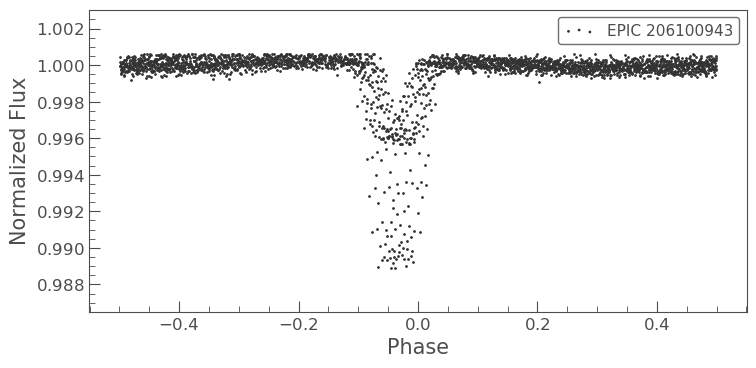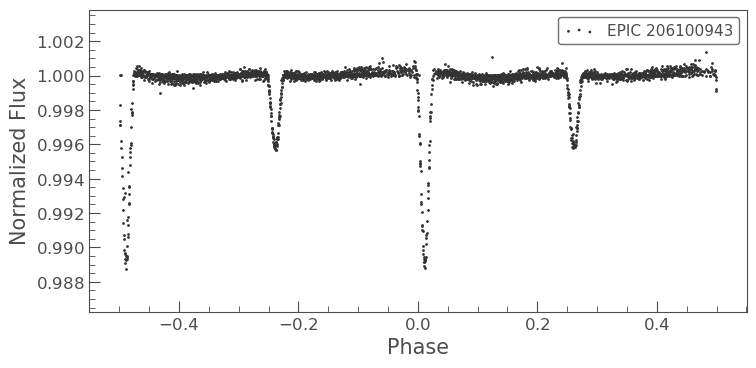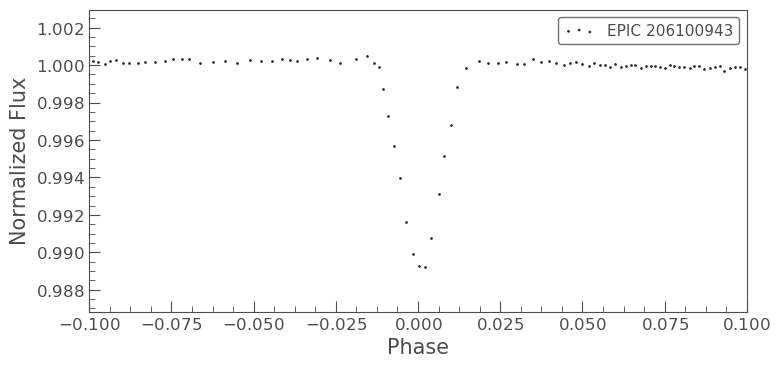Fig 7. – Top: LS unable to resolve primary and secondary transit (EPIC 206100943). Middle: BLS showing both transits for (EPIC 206100943). Bottom: BLS phase folded diagram for primary transit (EPIC 206100943).

5. Calculating planet parameters

After modelling the transit, it is possible to obtain information about the planet directly from the light curve and then calculate the planetary parameters after some basic assumptions. First determine equations that model the geometry of the system, this can be accomplished by calculated the total transit duration (when the dip begins to where it ends), full transit duration (the total time the planet is fully over the star) and then the planets radius. After obtaining equations to model the geometry of the system, along with Kepler’s third law and the stellar mass-radius relation it is possible to find a unique analytical solution with the parameters,  M∗ (Star mass), R∗ (Star radius), a (semi major axis), and i (orbital inclination).

Our basic assumptions if order for this model to work are;

• The planet orbit must circular in nature;

• Mp ≪ M∗ can be considered dark compared to the star;

• The stellar mass-radius relation is known;

• The light comes from a single star, rather than from two or more blended stars;

• The eclipses have flat bottoms which implies that the companion is fully superimposed on the central star’s disk;

• The period can be derived from the light curve (Shown above).

These assumptions are described by (S. Seager 2002), as well as the five equations that model the light curve. These equations are derived below with, transit depth (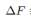) , total flux ( F ), planet radius ( Rp ), star radius (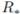) .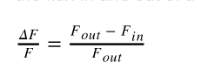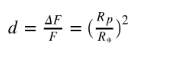Then finding the total transit duration (tT) and full duration (tF)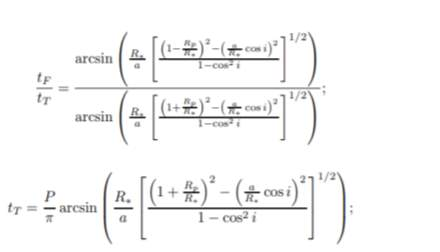Where (P = period)

Now that equations for transit geometry have be derived, it is possible to find the unique solution for the planet’s parameters. Firstly, the impact parameter b must be derived, which is defined as the distance between the centre of the star and the centre of the planet at the point in the orbit where two objects are most closely aligned. Then the ratio a/Radius of the star must be derived, both can be found from the last two equations using the fact that;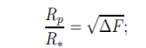The impact parameter and ratio are given by;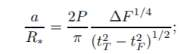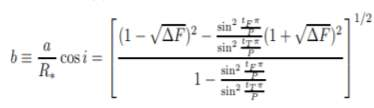Before calculating the parameters, an equation of the stellar density must be derived, this can be accomplished using Kepler’s third law and the previous ratio,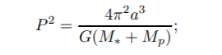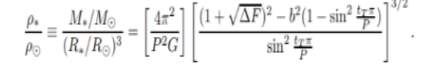The physical parameters are now given as follows, for derivation please see (S. Seager 2002).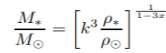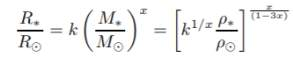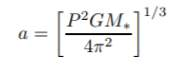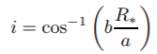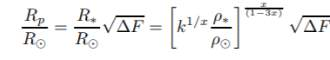Please note that planetary parameters can be seen in Fig 6. (Limb darkening diagram)

6. Modelling using calculated parameters

6.1 Batman parameters

Now using these equations, the planets parameters can be calculated, after which we can model the light curve using a library (batman), with a few assumptions.  The batman library takes parameters for orbital period, planet radius, semi major axis, orbital inclination, eccentricity, longitude of periastron, limb darkening model and limb darkening coefficients. For eccentricity which can be defined as a measurement of how much a planets orbit deviates from a perfect circle, I assumed a value of zero for simplicity, this can be assumed if we take the orbit to be a perfect circle. For longitude of periastron which can be defined as the longitude at which the nearest approach to the star would occur if the body’s orbit inclination were zero. I had no way of determining this value, so assumed a value of 90 (which is standard procedure for modelling in batman).

Limb darkening is the phenomenon where the edges of a star appear to be less bright then the centre due to the angle from which it is viewed. Limb darkening can be observed in the shape of the exoplanet transit as seen in the diagram below, this unique shape can be modelled using a number of different laws, in this report limb darkening is modelled using the quadratic limb darkening law which is described nicely in (D.K Sing 2010). Given below is the quadratic limb darkening equation, where c1 and c2 are limb darkening coefficients, μ = cos γ where γ is the angle between a line normal to the stellar surface and the line of sight of the observer.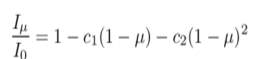Both constants can be found in (D.K Sing 2010), which gives coefficients based on the star’s effective temperature, which can be determined by looking at a star catalogue. All the other parameters that batman needs have been calculated as described above. The quadratic law for limb darkening is widely used to a high level of precision for modelling exotransit photometry using Monte-Carlo Markov-Chain (MCMC) techniques, since it allows for analytical calculation of light-curves with good computational efficiency (Mandel & Agol 2002).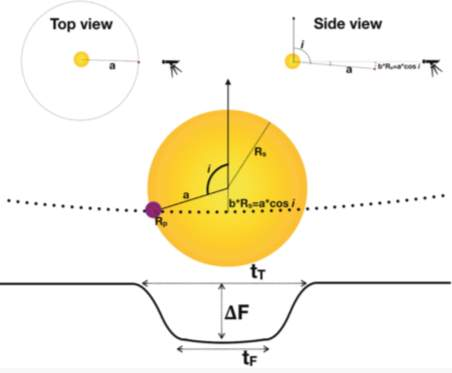Fig 8.- (Diagram for limb darkening) – We can see the wavelength-dependent decrease in specific intensity and the start and end of the transit

6.2 Accuracy of the quadratic limb darkening law

It has been shown that the depth of the transit is primarily determined by the ratio of the radius of the transiting exoplanet to the star. Limb darkening has an effect on the geometry of the transit, which will cause errors in calculating the planets true radius. This can be quite problematic as limb darkening not only modifies the shape of the transit light curve, but also significantly affects the true transit depth. One common approach is to model this effect with set parameters, but in recent studies it has been proven that limb darkening coefficients greatly vary from one author to the next due to discrepancies in the temperature region that is used. As I did not have enough time to continual update the coefficients I referred to (Sz. Csizmadia 2012), who investigate the inaccuracy caused by fixing the limb darkening coefficients by calculating the error caused by theoretical uncertainties. Focusing on the 3500−8000 K temperature range, because most of the transiting exoplanets have been detected around main sequence stars with such temperatures, from (Sz. Csizmadia 2012) error analysis I decided that (D.K Sing 2010) table of coefficients was the most suited to my research.

7.  Bayesian Statistical Inference

The goal of Bayesian inference is to make probability statements about unobserved quantities conditional on observed data (Gelman 2013). In the case of parameter estimation (model fitting), we want to infer the joint probability distribution for the model parameters given observational data and prior information. This probability distribution is called the posterior distribution (posterior), and it is obtained by updating a prior distribution (prior) with a sampling distribution (likelihood). For the first likelihood model, only two parameters were modelled for (period, phase). The simple likelihood model will work for just two parameters, when needed to take more parameters into account, a different method called Markov chain Monte Carlo (MCMC) can be used.

7.1 maximum likelihood estimators

The BLS function does not have analytical maximum likelihood estimators for all its parameters. Maximum likelihood estimation is a method that determines values for the parameters of a model. The parameter values are found such that they maximise the likelihood that the process described by the model produced the data that was actually observed. If the distribution from which the data was drawn is known, then it is possible to compute the probability or likelihood of the data. In order to calculate the maximum likelihood, it must be assumed that all the data points have been created independently of each other, then the likelihood is calculated by summing all the individual probabilities. Maximising our likelihood is similar to minimising the chi square. I used a package called Henrietta to calculate the likelihood for a simple model of two parameters (period, phase), this is accomplished as follows;

Firstly, a function that returns the model of the data that will be fitted is defined. In this case, the model is a function of two variables, the period and phase, whose values will be inferred. Two arrays are then defined one for periods and one for phase both of 1,000 possible periods/phases this range should be large enough to contain our true values. Chi-squared describes how good of a fit the model is to the data, defining chi square as,

where mt is the value of the model at time, ft is the value of the flux, and the sum is taken over the entire timeseries. For each pair of values, the program will compute the model and the chi-squared value of that model, the chi-squared is then stored in a two-dimensional array chisq, where chisq[i, j] corresponds to the chi-squared of the ith period and the jth phase. Once chi square has been computed the likelihood function can be plotted and then a Gaussian can be fitted to the likelihood so an uncertainty on each of the parameters can be obtained.

7.2 Markov chain Monte Carlo (MCMC)

Markov chain Monte Carlo (MCMC) is a method for modelling probabilities. A Markov Chain can be defined as a random process where a parameter value can move from one state to another with some underlying probability. A Markov Chain can be constructed to find the posterior distribution for model parameters, for the exoplanet’s parameters. As stated above our grid search won’t work as there are now too many possible combinations for the parameters. In order to model for all the parameters, I will attempt to create a Markov Chain, but first a few key ideas in Bayesian Statistics must be defined.

A prior distribution of a parameter can be defined as the probability distribution of the uncertainty of the parameter before the data is considered. Multiplying the prior distribution and the likelihood function together leads to the posterior distribution of the parameter, the posterior distribution is used to carry out all inferences. This means that, in Bayesian Statistics the posterior distribution encodes the information about the model parameters based on our prior and the likelihood from observations, where the likelihood can be defined as the probability that the observations follow from a given model evaluated at a given point in the model parameter space. We can obtain posterior probabilities, by integrating the posterior over all other parameters than the parameter of interest. Finally, different summary statistics can be derived to describe the posterior distributions concisely.

I used two libraries emcee and corner in order to construct my MCMC chains, where the chains are like “random walks” through a parameter space. This method creates samples that either accepts or rejects the move based on the posterior density ratios between the current and proposed locations. The location after the proposal is added to the chain. Markov chain Monte Carlo (MCMC) sampling is a fundamental tool for posterior estimation when we cannot sample from the posterior directly. In summary the code will formulate the likelihood, choose a prior, determine the posterior, search for the model parameters that maximize this and then quantify the uncertainty of the model parameter estimates.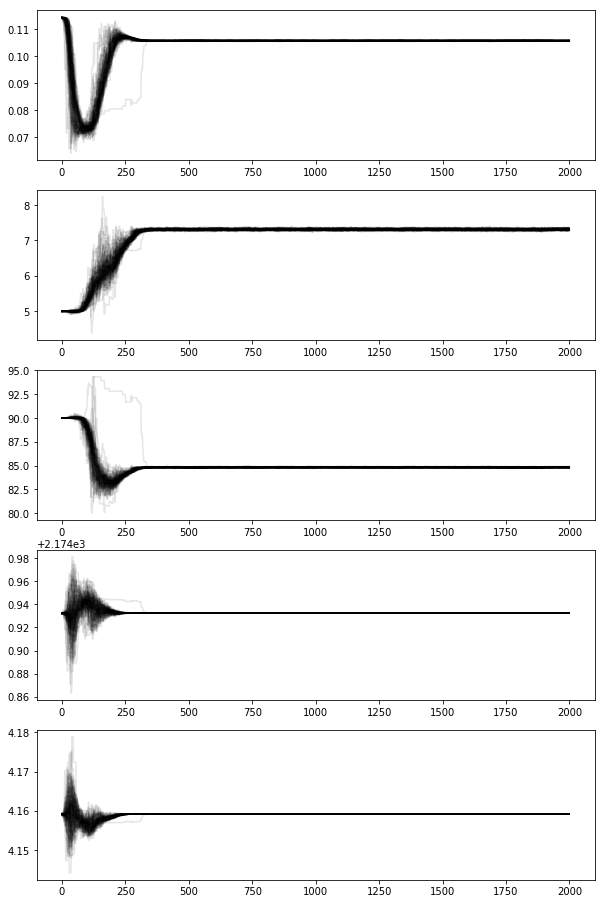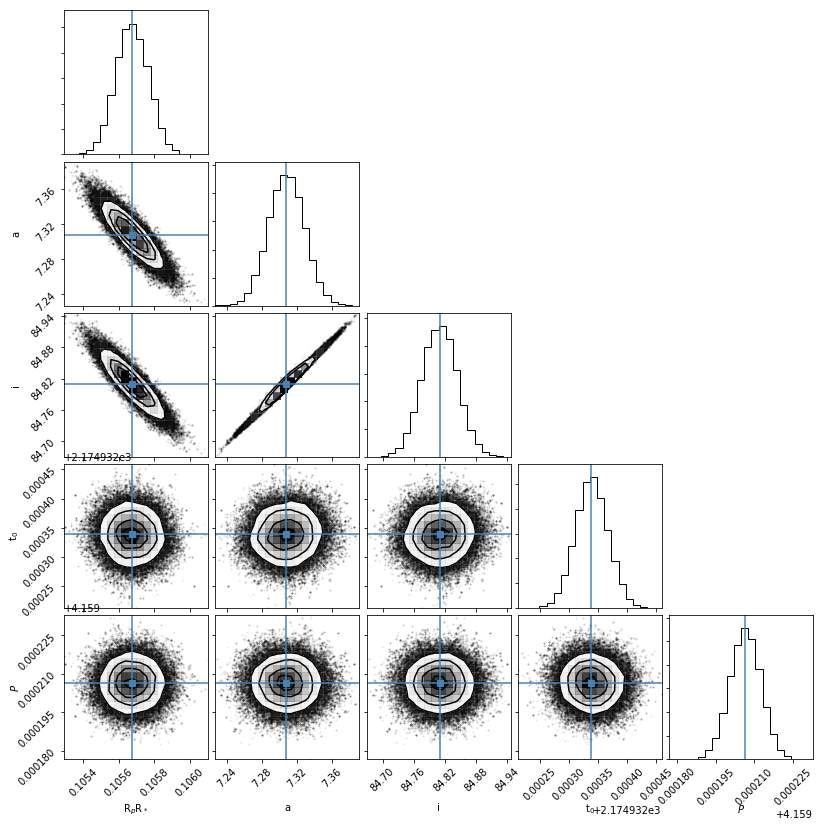Fig 9.- Top: Markov Chain for (EPIC 206103150), for planet parameters top to bottom ( $\mathit{Rp}{R}_{*}$

(radius planet, radius star), a (semi-major axis),  i (orbital inclination), ${t}_{o}$

(epoch),  P(period)).

Bottom:  posterior distributions of the planet’s parameters.

8. Results

#### Below all results for the orbital period are given in days. For each planet in NASAs exoplanet archives there is a given orbital period with a reference, in some cases where were multiple values given, in this case the first suggested values given was used. In order to calculate the values from the LS and BLS programs I used the programs that were created previously, I had to adjust ranges for the period and durations as needed for the fit. In order to verify my results, I used MAST, NASAs exoplanet archives, and the ExoFOP-K2 archive.

 K2 EPIC ID LS orbital period BLS orbital period NASA orbital period 206317286 1.249016 1.3125599 1.58252+0.00017-0.00018 206024342 5.915825 6.3837848 4.50756+0.00062-0.00060 206042996 4.926651 5.0161157 5.29711+0.00074-0.00070 206317286 1.326009 1.5230015 1.58252+0.00017-0.00018 211822797 16.701430 18.90318 21.169861+0.001725-0.001695 212297394 3.578932 3.6093543 2.28943±0.00019 212424622 14.50122 15.4582167 18.0983+0.0060-0.0058 212737443 11.027780 12.92100 13.6030±0.0013 246851721 5.7130270 6.1745779 6.180235±0.000014 206268299 17.802176 18.842900 19.5666±0.0033 201615463 3.2041090 3.6109345 3.80464+0.00091-0.00105 201833600 3.0981273 3.7103560 3.96151+0.00046-0.00051 211970147 8.567809 9.5091127 9.915615+0.001209-0.001195 216468514 2.906709 3.3890213 3.313959±0.000024 211442297 19.87723 21.987200 20.272615+0.000356-0.000343 212006344 1.956015 2.1804560 2.219282+0.000128-0.000130 247887989 2.801768 3.1209776 3.07133±0.00011 201227197 2.900817 3.6701566 3.705871 +0.000074-0.000076 211048999 4.800123 5.0128903 5.172191 +0.000201-0.000202 211906650 35.07234 43.903278 41.474219 +0.003288-0.004316 220621788 11.09823 12.089674 13.683256+0.001050-0.001100 206348688 15.0945 17.61235 18.286069 +0.005737-0.004564 210448987 4.9054 6.209123 6.102325+0.000390-0.000407 211077024 1.3987 1.423900 1.419604+0.000062-0.000060 211391664 8.9043 9.610943 10.136448+0.000609-0.000591 228733615 2.7030 3.309812 3.27108+0.00049-0.00050 228798746 2.3097 2.620987 2.69828±0.00022 228804845 2.4590 2.560234 2.86041±0.00061 219800881 11.907 12.92345 13.841901±0.001352 201092629 23.908 24.09788 26.81990+0.00245-0.00247 201102594 5.9876 6.390812 6.51389+0.00082-0.00083 201357643 9.8912 10.1208 11.89307+0.00065-0.00063 201386739 4.9875 5.29013 5.76918+0.00081-0.00082 228739306 6.6095 7.19940 7.17256+0.00148-0.00142 228748383 9.9345 11.9078 12.40900+0.00337-0.00284 216490365 2.9806 3.24560 3.484552+0.000031-0.000037 228748826 3.7340 4.21092 4.01457+0.00062-0.00057 228758778 8.9012 9.0921 9.30075+0.00332-0.00321 228763938 9.0564 11.097 13.81513+0.00461-0.00448 228809550 3.2109 3.5990 4.00167±0.00013 228894622 1.3903 1.7890 1.96417±0.00004 228968232 5.2309 5.6701 5.52011+0.00239-0.00289 228974324 1.3989 1.5908 1.60588+0.00014-0.00013 229617395 15.789 16.097 19.09210+0.00576-0.00633 229131722 12.908 14.908 15.48043+0.00332-0.00392 202083828 11.780 13.920 14.5662±0.0019 282911830 2.2305 2.5621 2.6266657±0.0000018 201498078 8.2360 9.0126 11.63344±0.00012 211682544 38.235 42.823 50.818947±0.000094 211713099 6.6230 7.9562 8.562421±0.000127 211816003 12.980 13.521 14.453533+0.001328-0.001360 211919004 7.8210 9.8012 11.716332+0.001172-0.001198 212008766 12.501 13.901 14.129714+0.002102-0.001876 212130773 16.701 18.903 18.718269+0.003153-0.003008 212357477 5.6690 6.2091 6.326763+0.000355-0.000361 212418133 2.9883 3.2490 3.334966+0.000701-0.000859 212782836 5.6933 6.6193 7.122605+0.001647-0.001601 206318379 1.8034 2.2840 2.2604455±0.0000009 216494238 16.702 18.256 19.895202+0.000774-0.000753 220522262 8.2378 8.6125 8.687721+0.000151-0.000150 220554210 3.7922 4.2386 4.169836+0.000279-0.000300 220629489 1.7643 1.8720 1.921036+0.000051-0.000053 247267267 4.5106 4.6195 4.795069±0.000086 211089792 2.9801 3.1902 3.258824±0.000009 220501947 3.8104 4.1034 4.024867±0.000015 210957318 3.2015 3.8905 4.098492 +0.000027-0.000028 204129699 0.9451 1.1945 1.257850±0.000002 205071984b 5.9805 7.0231 8.991828+0.000083-0.000084 205071984c 16.984 18.237 20.66186+0.00102-0.00098 205071984d 25.790 27.130 31.7142+0.0011-0.0010 205071984e 3.9042 4.2053 4.34882+0.00069-0.00075 205117205 4.6209 4.9245 5.424865+0.000035-0.000031 212110888 1.9354 2.1845 2.995633±0.000008 201549860 5.2981 5.4021 5.608352+0.000335-0.000338 206247743 4.0732 4.3012 4.60497±0.00077 201208431 9.7601 9.9065 10.003417+0.001017-0.000996 201155177 6.0348 6.2987 6.68796±0.00093 201205469 3.2590 3.4522 3.471341+0.000163-0.000161 201295312 4.9021 5.3523 5.656304 +0.000366-0.000323 201345483 1.8349 1.9336 1.729257±0.000008 201403446 17.012 18.451 19.154540 +0.002849-0.002764 201647718 25.318 29.518 31.6372±0.0088 201677835 18.724 19.045 20.5065±0.0035 201690311 2.9076 2.8923 2.77065±0.00015 206103150 4.1695 4.1560 4.160710000 ± 0.000380000 228735255 6.6207 6.5631 6.569180000 ± 0.000040000 246067459 3.1085 3.2073 3.204660000 ± 0.000030000

8.1 Discussion of Results

As seen in the table of results the BLS algorithm is by far the most precise algorithm, both algorithms tend to generally underestimate the given value. Furthermore, planets with shorter periods are modelled more accurately then planets with longer parameters, planets of larger masses are also detected with greater ease as the dip observed is much larger. Lastly systems with multiple planets tend to be modelled less accurately then systems with only one planet. Light curves that still had noise or unusual spikes caused inaccurate measuring of the period, I have outlined how I would fix this in future, in section 10 of this report. As stated above NASA’s archive were used along with ExoFop- K2 archive which provides much more information then NASA’s archives, for example some exoplanets that have been discovered, as well as pulsating, or eclipsing binary stars that can be found in the k2 light curves. I located two possible pulsating stars EPIC 201885041 and EPIC 206100942 what weren’t given in NASA’s archive but can be found in ExoFop- K2 archive. Furthermore, the planet found in EPIC 246067459 wasn’t given in NASA’s archive, I found find no reason for this exclusion.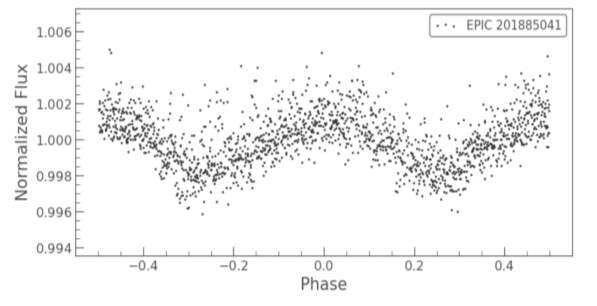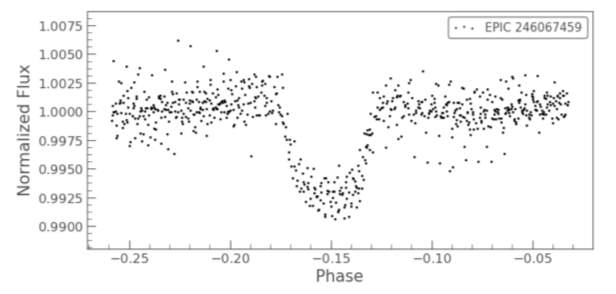Fig 10- Top: Possible pulsating star (EPIC 201885041). Bottom: exoplanet excluded from NASA’s archive (EPIC 246067459).

9. Conclusion

After processing and searching data from the Kepler space telescope in its k2 extended mission for transiting planet candidates, I identify 84 planet candidates. In summary the code removes, poor quality data points, removes outliers, removes long term trends and removes infinite values. Then two algorithm LS and BLS are used to detect the transit and fit for a period, after which the light curve is folded and binned. Then the planets parameters are calculated, and the curve is refitted while modelling for limb darkening. After we can calculate posterior distributions for the planet’s parameters. This research project suffered from errors caused by assumptions that were made in order to simplify problems and factors that weren’t considered in the scope of this research project, which will be discussed in that order.

Find Out How UKEssays.com Can Help You!

Our academic experts are ready and waiting to assist with any writing project you may have. From simple essay plans, through to full dissertations, you can guarantee we have a service perfectly matched to your needs.

Firstly, before searching for transits in the light curve we can see what appears to be left over systematic error that hasn’t been removed by SFF in some of the light curves, this could lead to inaccurate calculations for the orbital period. In future a more thorough SFF process should be applied. Secondly there is not a list of calculated planet parameters, therefore I could not compare results to given values for all the detected planets, as NASA’s currently doesn’t provide that information. I was unable to find a large enough data base that include most of my detected planets. Furthermore, setting eccentricity, longitude of periastron, limb darkening model and limb darkening coefficients for modelling caused some inaccuracies, for more accurate results this parameter would have to be update as necessary. Lastly, calculated posterior distributions for the planet’s parameters mostly suffered from lack of valid points, this error would have to be investigated in future research.

Furthermore, there are several factors that the code doesn’t consider, which could cause inaccuracies in fitting. Firstly, my Savitzky-Golay filter oversmoothed the transits, in future I would try to find a method of removing long term trends without this error. Secondly for long-period planets, the assumption of a circular orbit is in general not justified, assuming the eccentricity value is zero, makes the orbital period estimates artificially tight as shown in (Andrew Vanderburg 2016). Implementing non-zero eccentricity on the photometric light curve model would be an interesting way to investigate how the geometry of the transit changes with eccentricity. Lastly, parameter values that were used for modelling the transit with batman should have been experiment with for thoroughness. Overall the program detects and models for exoplanet transit quite accurately, but regrettably there was not enough time to test the program on all the data taken by the k2 mission. I would like to thank my supervisor Dr. Nicholas Devaney and acknowledge the efforts of the entire Kepler/k2 team.

10. Future Research

In future research projects there are several ways which the current code could be improved, there are also many other interesting areas of research that are currently outside the scope of this project. Firstly, in order to reduces noise further and improve transit modelling, I could adjust the pipeline used for SFF as necessary. Hence it may be possible to improve the photometric precision of individual stars, when objects of interest are revealed using the MAST pipeline. Another data process method that could yield higher precision is modelling for low-frequency variability using a Markov Chain Monte Carlo algorithm with an affine invariant ensemble sampler (Goodman & Weare 2010).

Furthermore, a false positive system both for instrumental false positives and astronomical false positives would be worth developing. An instrumental false positive test would be an effect way to test if the SFF process was thorough enough. Astrophysical phenomena other than transiting exoplanets, including eclipsing binary star and background sources in the photometric aperture, can cause the detection of a transit. In my research project I identified these situations manually and confirm using an exoplanet archive. The vespa software (Morton 2012, 2015) is commonly used to evaluate the false-positive probability for planetary candidates and could be used in future research.

Another interesting area to investigate would be the dynamical stability of the system of a multiple planet system. In order to do this, simulation of the orbits would be created using a program like Mercury6, to assess the resonance state of the system. Furthermore, other factors such as density and atmosphere can be investigated. Firstly, the density can give clues about the planet’s composition and whether or not it might have an atmosphere. It would be interesting to comparing the spectrum from the host star, and the spectrum of an exoplanet, this would give information on the composition of a planet’s possible atmosphere.

Currently cutting-edge research is underway as, many teams are attempted to confirm the first exomoon. David Kipping and graduate student Alex Teachey using both a photometry detection method and the Hubble Space Telescope have proposed the possibility of a moon orbiting Kepler-1625b. Unfortunately, this hasn’t been confirmed yet as the exomoons signal is very small compared to the planets, furthermore differences in data reduction by another research team suggest that a basic planet model fits the data with a higher degree of accuracy.

11. References

• Vanderburg, A., Becker, J., Kristiansen, M., Bieryla, A., Duev, D., & Jensen-Clem, R. et al. (2016). FIVE PLANETS TRANSITING A NINTH MAGNITUDE STAR. The Astrophysical Journal827(1), L10. doi: 10.3847/2041-8205/827/1/l10
• Becker, J., Vanderburg, A., Adams, F., Rappaport, S., & Schwengeler, H. (2015). WASP-47: A HOT JUPITER SYSTEM WITH TWO ADDITIONAL PLANETS DISCOVERED BY K2. The Astrophysical Journal, 812(2), L18. doi: 10.1088/2041-8205/812/2/l18
• Seager, S., & Mallen‐Ornelas, G. (2003). A Unique Solution of Planet and Star Parameters from an Extrasolar Planet Transit Light Curve. The Astrophysical Journal585(2), 1038-1055. doi: 10.1086/346105
• Vanderburg, A., & Johnson, J. (2014). A Technique for Extracting Highly Precise Photometry for the Two-WheeledKeplerMission. Publications Of The Astronomical Society Of The Pacific126(944), 948-958. doi: 10.1086/678764
• Pál, A. (2008). Properties of analytic transit light-curve models. Monthly Notices Of The Royal Astronomical Society, 390(1), 281-288. doi: 10.1111/j.1365-2966.2008.13723.x
• Heller, R., Hippke, M., & Rodenbeck, K. (2019). Transit least-squares survey. Astronomy & Astrophysics627, A66. doi: 10.1051/0004-6361/201935600
• Livingston, J., Crossfield, I., Petigura, E., Gonzales, E., Ciardi, D., & Beichman, C. et al. (2018). Sixty Validated Planets from K2 Campaigns 5–8. The Astronomical Journal156(6), 277. doi: 10.3847/1538-3881/aae778
• Mayo, A., Vanderburg, A., Latham, D., Bieryla, A., Morton, T., & Buchhave, L. et al. (2018). 275 Candidates and 149 Validated Planets Orbiting Bright Stars in K2 Campaigns 0–10. The Astronomical Journal155(3), 136. doi: 10.3847/1538-3881/aaadff
• Vanderburg, A., Latham, D., Buchhave, L., Bieryla, A., Berlind, P., & Calkins, M. et al. (2016). PLANETARY CANDIDATES FROM THE FIRST YEAR OF THE K2 MISSION. The Astrophysical Journal Supplement Series222(1), 14. doi: 10.3847/0067-0049/222/1/14
• Sing, D. (2010). Stellar limb-darkening coefficients for CoRot and Kepler. Astronomy And Astrophysics510, A21. doi: 10.1051/0004-6361/200913675
• Transit Light Curve Tutorial. (2019). Retrieved 12 August 2019, from https://www.cfa.harvard.edu/~avanderb/tutorial/tutorial3.html
• christinahedges/k2torials. (2019). Retrieved 12 August 2019, from https://github.com/christinahedges/k2torials
• Lightkurve docs — Lightkurve. (2019). Retrieved 12 August 2019, from https://docs.lightkurve.org/
• Tutorial — batman 2.4.6 documentation. (2019). Retrieved 12 August 2019, from https://www.cfa.harvard.edu/~lkreidberg/batman/tutorial.html
• PyKE: Kepler, K2 & TESS Data Analysis Tools — PyKE 3.0.0 documentation. (2019). Retrieved 12 August 2019, from http://pyke.keplerscience.org/index.html
• Astropy. (2019). Retrieved 12 August 2019, from https://www.astropy.org/
• Limb darkening. (2019). [Image]. Retrieved from http://Transit Photometry as an Exoplanet Discovery Method
• Starr, M. (2019). The First Exomoon Ever Detected in Space Might Not Actually Exist, Scientists Say. Retrieved 13 August 2019, from https://www.sciencealert.com/the-first-known-exomoon-is-called-into-question-in-follow-up-studies
• NASA Exoplanet Archive. (2019). Retrieved 13 August 2019, from https://exoplanetarchive.ipac.caltech.edu/
• STScI | Mikulski Archive for Space Telescopes (MAST) Portal. (2019). Retrieved 13 August 2019, from https://mast.stsci.edu/portal/Mashup/Clients/Mast/Portal.html
• ExoFOP-K2. (2019). Retrieved 13 August 2019, from https://exofop.ipac.caltech.edu/k2/index.php
• Csizmadia, S., Pasternacki, T., Dreyer, C., Cabrera, J., Erikson, A., & Rauer, H. (2012). The effect of stellar limb darkening values on the accuracy of the planet radii derived from photometric transit observations. Astronomy & Astrophysics549, A9. doi: 10.1051/0004-6361/201219888
• Mandel, K., & Agol, E. (2002). Analytic Light Curves for Planetary Transit Searches. The Astrophysical Journal580(2), L171-L175. doi: 10.1086/345520
• Goodman, J., & Weare, J. (2010). Ensemble samplers with affine invariance. Communications In Applied Mathematics And Computational Science, 5(1), 65-80. doi: 10.2140/camcos.2010.5.65

## Cite This Work

To export a reference to this article please select a referencing stye below:

View all

## DMCA / Removal Request

If you are the original writer of this essay and no longer wish to have your work published on UKEssays.com then please:

Related Services

Related Lectures

Study for free with our range of university lectures!Freelance Writing Jobs

Looking for a flexible role?
Do you have a 2:1 degree or higher?

Study Resources

Free resources to assist you with your university studies!